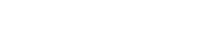# Certified Automation Professional (CAP) Question

• CAP

## CAP question

For a resistance temperature device, the term “alpha” in the equation below is typically called?

R = R0 ( 1 + α ΔT )

A. temperature coefficient of resistance
B. temperature inverse scaling factor
C. RTD normalization constant
D. Coefficient of heat transfer

The answer is A, “temperature coefficient of resistance.” Resistance temperature device (RTD) elements can be characterized by their temperature coefficient of resistance (TCR). This constant is also referred to as the alpha constant for the RTD. RTDs can be manufactured from different metals, and each RTD specification has its own alpha value. For example, the IEC 60751 standard defines the platinum Pt100 RTD as having a resistance of 100 ohms at 0°C with an alpha coefficient of α = 0.00385. Using the equation in the problem statement with R0 = 100 ohms, a Pt100 RTD will change resistance by 0.385 ohms/°C.

Reference: Sands, Nicholas P. & Verhappen, Ian, A Guide to the Automation Body of Knowledge, Third Edition, ISA Press, 2019.